Test wiedzy na operatora reaktora BWR
Test składa się z 50 pytań jednokrotnego wyboru, aby zaliczyć test należy uzyskać przynajmniej 80% poprawnych pytań (40). Test jest zgodny z wymaganiami stawianymi przez amerykański dozór jądrowy, Operator Licensing Examination Standards for Power Reactors, NUREG-1021, Rev. 11.
Test w języku angielskim.

Powodzenia,
Rafał
1. Refer to the drawing of an open system with subcooled water flowing through valves A, B, C and D (see figure below). All valves are initially 50 percent open. The inlet pressure to valve A is constant at 60 psia. The initial steady-state inlet and outlet pressures for valve B are as follows: I) Inlet pressure = 50 psia II) Outlet pressure = 35 psia. After a single valve operation, the current steady-state inlet and outlet pressures for valve B are as follows: I) Inlet pressure = 48 psia II) Outlet pressure = 36 psia. Which one of the following valve operations could be responsible for the difference between the initial and current steady-state inlet and outlet pressures for valve B? *
1 point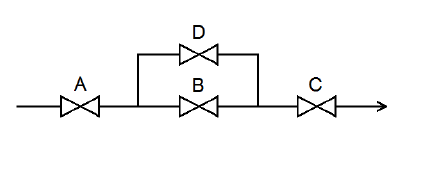2. Refer to the drawing of four air-operated valves (see figure below). Note: The valve actuators may be shown with or without air pressure applied.Which valves are currently shown in their failed (i.e., no air pressure applied to the actuator) positions? *
1 point3. A typical check valve is designed to... *
1 point
4. A steam flow measuring instrument uses density compensation and square root extraction to convert the differential pressure across the flow element to flow rate in lbm/hr. The purpose of density compensation in this flow measuring instrument is to convert __________ into __________. *
1 point
5. Refer to the drawing of a reactor vessel differential pressure (D/P) level detection system that was calibrated at 1,000 psia (see figure below). A reactor vessel cooldown has resulted in a decrease in reactor vessel pressure from 1,000 psia to 500 psia over several hours. Without density compensation of the level instrumentation, at the end of the cooldown, reactor vessel level indication would indicate __________ than actual level because the density of the water in the __________ has changed significantly. (Assume the reference leg does not flash to steam.) *
1 point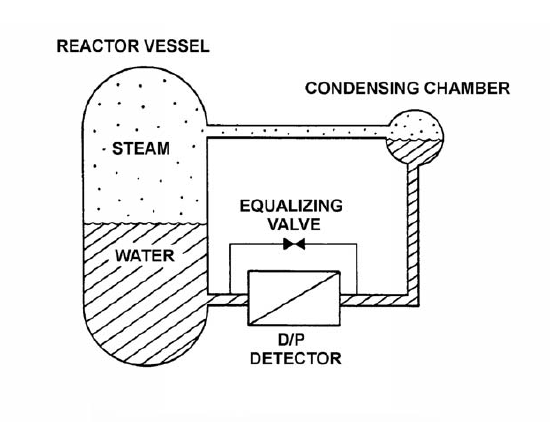6. Refer to the simplified drawing of a control rod position detector circuit (see figure below). A magnet on the control rod extension (or drive) shaft sequentially closes individual reed switches mounted vertically adjacent to the control rod drive housing. A constant +5 DC volts is supplied to the input of the resistor network at resistor R1. A control rod is initially fully inserted such that all reed switch contacts are open; then the rod is withdrawn until reed switch contact S1 is closed. Compared to the initial circuit currents, the current through resistor R5 after the rod withdrawal will be __________; and the output current of the resistor network to the amplifier will be __________. *
1 point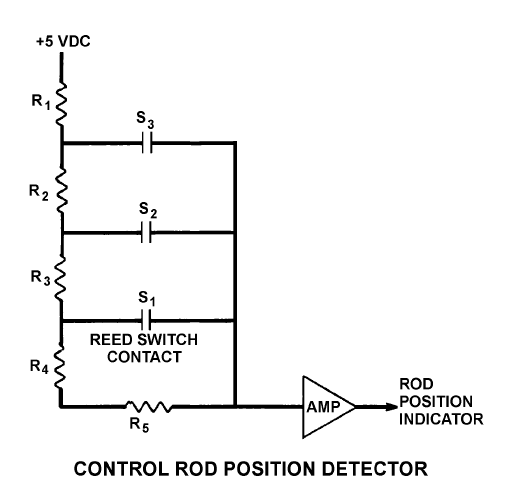7. Following a loss-of-coolant accident, a reactor scram resulted. The source range monitors (SRMs) were inserted and are currently positioned in a voided region of the core. If the SRMs are subsequently repositioned to a water-filled region of the core, the indicated count rate will __________; primarily due to a __________ in the water-filled region of the core. *
1 point
8. A vertical safety valve with a 3-inch diameter disk has a spring applying 1000 lbf to the top of the valve disk in opposition to system pressure. Which one of the following is the approximate system pressure at which the safety valve will begin to open? *
1 point
9. A main steam system uses a combination of safety and relief valves for overpressure protection. Which one of the following describes a major design consideration for installing both types of valves in the same system? *
1 point
10. Refer to the drawing of a cooling water system in which both centrifugal pumps A and B are operating (see figure below). An operator stops pump B, but the pump B check valve fails to close. In comparison to normal operation with only pump A running, operation with the failed pump B check valve will result in pump A flow rate being __________ than normal; and heat exchanger flow rate being __________ than normal. *
1 point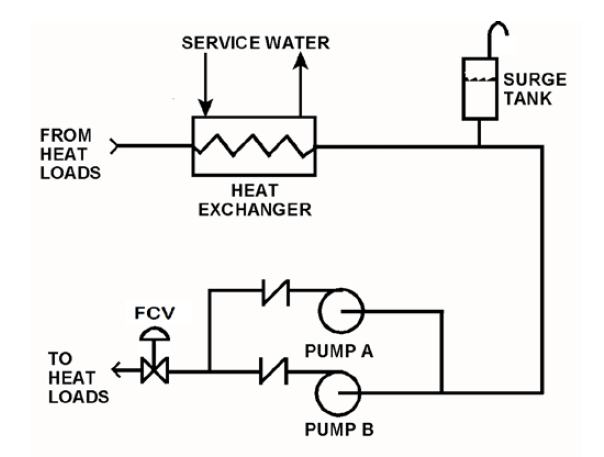11. Two common types of check valves used in nuclear power plants are... *
1 point
12. What happens to the pressure and velocity of water as it passes through a venturi? *
1 point
13. Refer to the drawing of a reactor vessel (RV) differential pressure (D/P) level detection system (see figure below) that was recently calibrated at normal operating conditions. With the reactor shut down, RV pressure was inadvertently decreased from 1000 psig to 500 psig in 5 minutes due to operator error. RV pressure was stabilized at 500 psig, but during the pressure decrease a small amount of water in the condensing chamber flashed to steam. Assume the reference leg water remained subcooled, except for the small amount of water that flashed to steam in the condensing chamber. As a result of the small loss of condensing chamber water, RV level will indicate __________ than actual level; and as the condensing chamber refills, indicated level will __________. *
1 point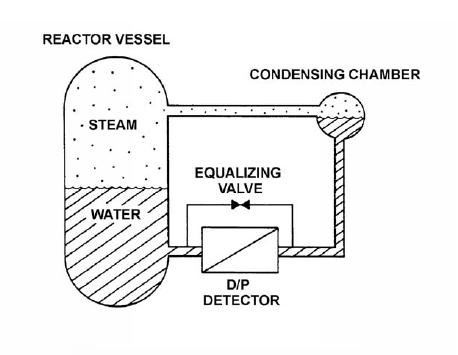14. Gamma radiation contributes to the output of a fission chamber mainly by interacting with the... *
1 point
15. Fission chamber detectors are used to monitor reactor power/neutron level in a shutdown reactor as well as a reactor operating at rated power (and all power levels in between). At what power level(s) is it necessary to compensate the output of the fission chamber detectors for gamma interactions with the detectors and why? *
1 point
16. Which one of the following lists the two types of gas-filled radiation detectors whose outputs will be least affected by a small variation (±10 volts) in the voltage applied to the detectors? (Assume the applied voltage remains within normal range.) *
1 point
17. The difference between the setpoint in an automatic controller and the steady-state value of the controlled parameter is called... *
1 point
18. Refer to the drawing of a water storage tank with a level control system (see figure below). The tank water level is being automatically controlled at 50 percent by a proportional-integral (PI) controller that positions the drain valve. Tank water level is currently stable with 500 gpm entering the tank and the drain valve is 50 percent open. Tank inlet flow rate suddenly increases to 700 gpm and remains constant. When tank water level stabilizes, level will be __________; and the drain valve position will be __________. *
1 point19. Refer to the drawing of a lube oil temperature control system (see figure below). The temperature control valve is currently 50 percent open.If the cooling water inlet temperature decreases, the temperature controller will position the temperature control valve more __________, causing cooling water differential temperature through the heat exchanger to __________. *
1 point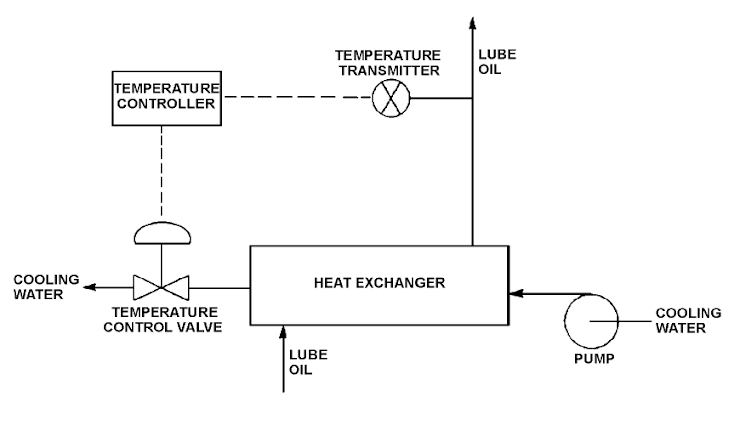20. Which one of the following changes in nuclear power plant status will bring the reactor recirculation system closer to the condition in which the recirculation pump will cavitate? *
1 point
21. Refer to the drawing of a cooling water system and the associated pump/system operating curves (see figure below). Pumps A and B are identical single-speed centrifugal pumps and initially only pump A is operating. Pump B is then started. After the system stabilizes, system flow rate will be...
1 point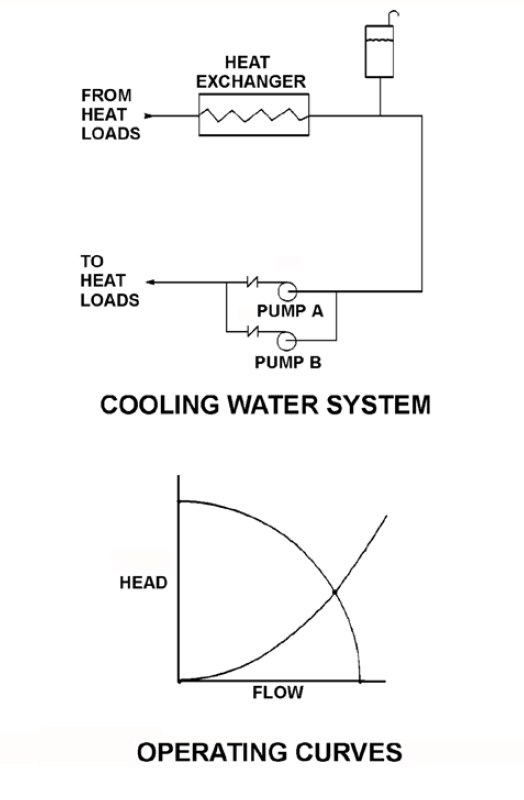22. Which one of the following will provide the first motor protection against electrical damage caused by gradual bearing degradation? *
1 point
23. Which one of the following types of neutrons has an average neutron generation lifetime of 12.5 seconds? *
1 point
24. Which one of the following conditions describes a reactor that is exactly critical? *
1 point
25. Which one of the following defines K-excess? *
1 point
26. The shutdown margin (SDM) upon full insertion of all control rods following a reactor scram from full power is __________ the SDM immediately prior to the scram. *
1 point
27. One hour ago, a reactor scrammed from steady-state 100 percent power due to an instrument malfunction. All systems operated normally. Given the following absolute values of reactivities added since the scram, assign a (+) or (–) as appropriate and choose the current value of core reactivity. I) Xenon = ( ) 1,0 %ΔK/K II) Fuel temperature = ( ) 2,0 %ΔK/K III) Control rods = ( ) 14,0 %ΔK/K IV) Voids = ( ) 3,0 %ΔK/K *
1 point
28. If reactor power changes from 10^-(5) percent to 10^(-6) percent in 5 minutes, the average reactor period is:
1 point
29. Reactor power was increased from 20 percent to 30 percent in one hour using only control rod withdrawal. Which one of the following describes the response of void fraction during the power increase? *
1 point
30. Initially, a nuclear power plant is operating at steady-state 100 percent power and 100 percent core flow rate. Then, reactor power is reduced to 90 percent by inserting control rods. (Assume that recirculation pump speed and valve positions do not change.) What is the effect of the power reduction on core flow rate? *
1 point
31. Reactors A and B are identical and have operated at 100 percent power for six months when a reactor scram occurs simultaneously on both reactors. All control rods fully insert, except for one reactor B control rod that remains fully withdrawn. Which reactor, if any, will have the longer reactor period five minutes after the scram, and why? *
1 point
32. Which one of the following increases in void fraction would produce the greatest amount of negative reactivity? *
1 point
33. Reactors A and B are identical except that reactor A has an effective delayed neutron fraction of 0,007 and reactor B has an effective delayed neutron fraction of 0,006. Initially, both reactors are critical at 1,0*10^(-8) percent power when +0,1 %ΔK/K is simultaneously added to both reactors. Five minutes after the reactivity additions, reactor _____ will be at the higher power level; and reactor _____ will have the shorter period. *
1 point
34. A reactor startup is in progress following a one-month shutdown. Upon reaching criticality, the operator establishes a positive 80-second period and stops control rod motion.After an additional five minutes, reactor power will be __________; and reactor period will be __________. (Assume reactor power remains below the point of adding heat.) *
1 point
35. A reactor startup is being performed by adding equal amounts of positive reactivity and waiting for neutron population to stabilize. As the reactor approaches criticality, the numerical change in stable neutron population resulting from each reactivity addition will __________; and the time required for the neutron population to stabilize after each reactivity addition will __________. *
1 point
36. A reactor startup is in progress with a current Keff of 0,95 and a stable source range count rate of 120 cps. Which one of the following stable count rates will occur when Keff becomes 0,98? *
1 point
37. Refer to the drawing of two water storage tanks with four differential pressure (D/P) level detectors (see figure below). The tanks are identical and are being maintained at 2 psig overpressure, 60°F, and the same constant water level. The tanks are located within a sealed containment structure that is being maintained at standard atmospheric pressure. All level detectors have been calibrated and are producing the same level indication.If a ventilation malfunction causes the containment structure pressure to decrease to 13 psia, which detectors will produce the highest level indications? *
1 point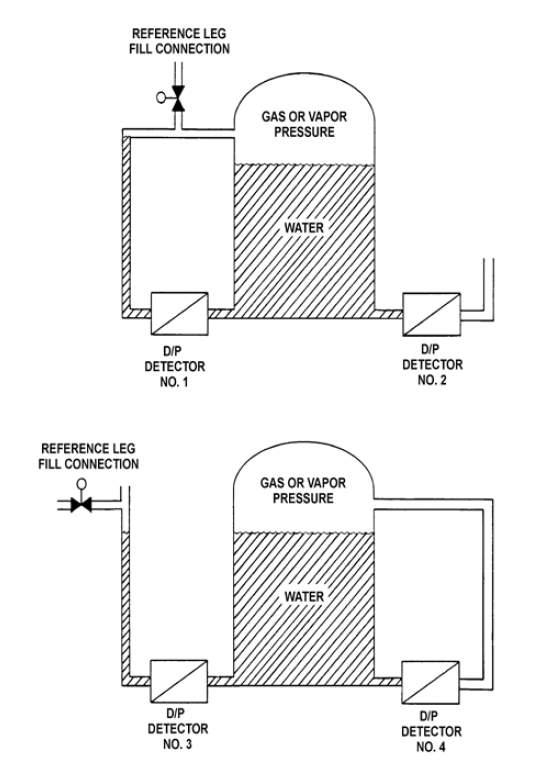38. For which of the following ideal processes, if any, is the steam inlet enthalpy equal to the steam outlet enthalpy? (Assume horizontal fluid flow in each process.) (A) Dry saturated steam flowing through a pressure reducing valve. (B) Dry saturated steam flowing through a fixed convergent nozzle. *
1 point
39. Refer to the drawing of a simple Rankine cycle shown on a Temperature-Entropy (T-S) diagram (see figure below). The starting point for the numbers on the diagram was chosen at random.Note: A simple Rankine cycle does not include condensate/feedwater heating, turbine exhaust moisture removal, or steam reheat.The sequence of numbers that represents the total heat added in the reactor vessel is __________; and the sequence of numbers that represents the total heat rejected in the main condenser is __________. *
1 point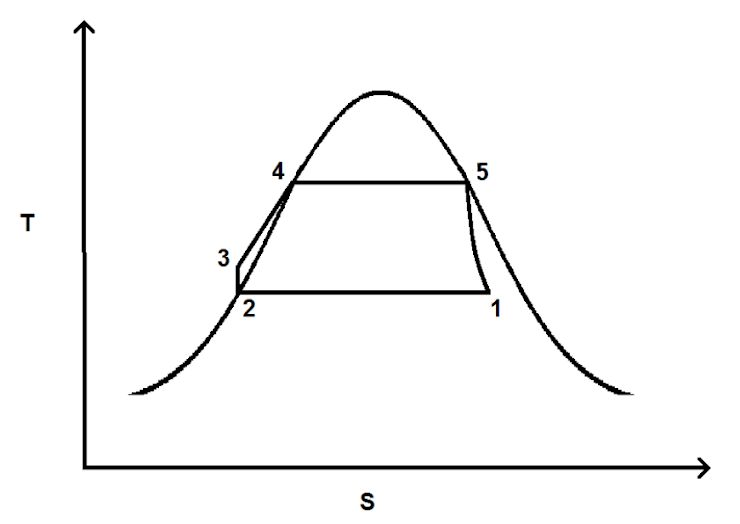40. Refer to the drawing of a lube oil heat exchanger (see figure below). The lube oil heat exchanger is in operation when the cooling water flow rate is increased to twice its former value. Which one of the following will increase as a result? *
1 point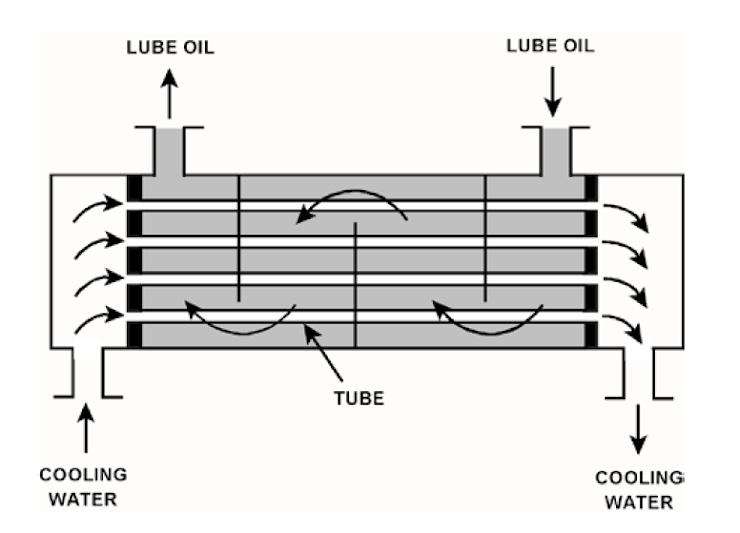41. Refer to the drawing of a pool boiling curve (see figure below). In which region(s) of the curve does a reactor normally operate to transfer heat from the fuel cladding to the coolant at 100 percent power? *
1 point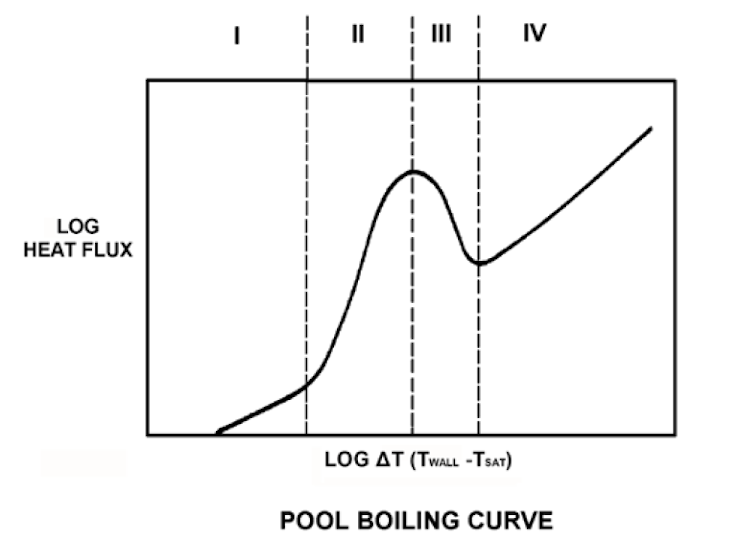42. Refer to the drawing of a hypothetical fuel bundle coolant channel (see figure below). For the hypothetical fuel bundle coolant channel shown below, at what point along its length does transition boiling begin? *
1 point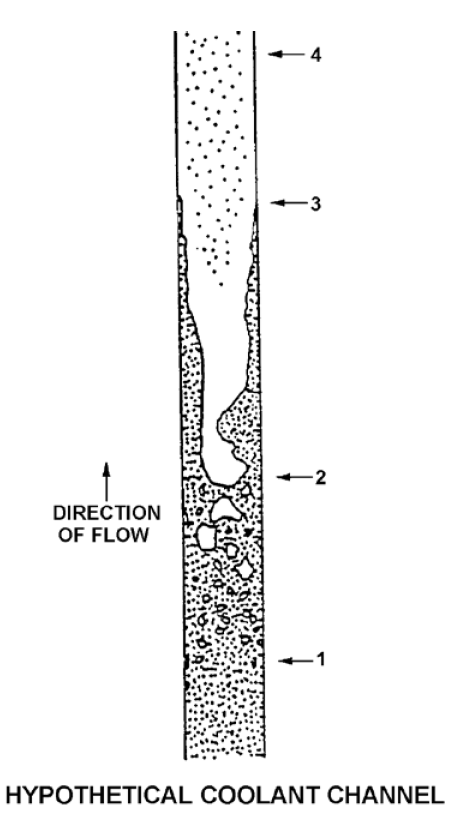43. Refer to the drawing of a core recirculation jet pump (see figure below). During normal operation, the lowest pressure will exist at point _____; and the highest velocity will occur at point _____. *
1 point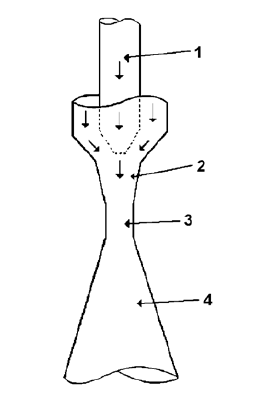44. The radial peaking factor for a fuel bundle is expressed mathematically as... *
1 point
45. A BWR core consists of 30 000 fuel rods. Each fuel rod has an active length of 12 feet. The core is producing 1 800 MW of thermal power. If the total peaking factor for a node is 1,6, what is the maximum local linear power density being produced in the node? *
1 point
46. The ratio of the highest fuel pin heat flux in a node to the average fuel pin heat flux in the same node is called the __________ peaking factor. *
1 point
47. Which one of the following is the most probable location for superheated steam in a boiling water reactor steam cycle that uses moisture-separator reheaters? *
1 point
48. What effect will occur if heat is removed from water that is in a subcooled condition? *
1 point
49. A nuclear power plant is operating at 80 percent power with 5°F of condensate depression in the main condenser. If the condensate depression decreases to 2°F, the steam cycle thermal efficiency will __________; and the condensate pumps will operate __________ cavitation. *
1 point
50. In a reactor operating at 100 percent power, reactor pressure suddenly increases. Which one of the following is the most limiting thermal limit for this situation? *
1 point